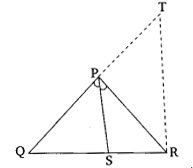# In the given figure, $PS$ is the bisector of $\angle QPR$ of $‚àÜPQR$. Prove that $\frac{QS}{SR}=\frac{PQ}{PR}$"

Given:

$PS$ is the bisector of $\angle QPR$ of $∆PQR$.

To do:

We have to prove that $\frac{QS}{SR}=\frac{PQ}{PR}$

Solution:Draw $RT \| PS$,meeting $QP$ produced at $T$.
$PS \| RT$
This implies,
$\angle 2=\angle 3$             (Alternate angles)
$\angle 1=\angle 4$        (Corresponding angles)
$\angle 1 = \angle 2$       (AD is the bisector of $\angle A$)
Therefore,
$\angle 3 =\angle 4$
This implies,
$PT=PR$
In $\triangle QRT$,
$\frac{QS}{SR}=\frac{PQ}{PT}$
$\frac{QS}{SR}=\frac{PQ}{PR}$

Hence proved.